American Journal of Applied Mathematics
Volume 3, Issue 3-1, June 2015, Pages: 6-13

General Solution for Free Convection of Viscous Fluid Near an Infinite Isothermal Vertical Plate that Applies a Shear Stress to the Rotating Fluid

M. A. Imran1, *, Shakila Sarwar2, D. Vieru3, M. Nazar2, 4

1Department of Mathematics, University of Management and Technology, Lahore, Pakistan

2Department of Mathematics, Bahauddin Zakariya University, Multan, Pakistan

3Department of Theoretical Mechanics, Technical University of Iasi, Iasi, Romania

4Department of Mathematics, Wenzhou Kean University, Wenzhou, China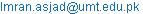(M. A. Imran)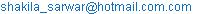(S. Sarwar)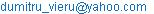(D. Vieru)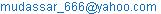(M. Nazar)

M. A. Imran, Shakila Sarwar, D. Vieru, M. Nazar. General Solution for Free Convection of Viscous Fluid Near an Infinite Isothermal Vertical Plate that Applies a Shear Stress to the Rotating Fluid. American Journal of Applied Mathematics. Special Issue: Proceedings of the 1st UMT National Conference on Pure and Applied Mathematics (1st UNCPAM 2015). Vol. 3, No. 3-1, 2015, pp. 6-13. doi: 10.11648/j.ajam.s.2015030301.12

1. Introduction

The study of free and forced convection flow past a vertical plate have drawn attention of many researchers considering different sets of thermal conditions at the boundary of the plate, due its industrial and technological applications. Gupta et al.  studied free convection flow past a linearly accelerated vertical plate in the presence of a viscous dissipative fluid, by using the perturbation method. Kafousias and Raptis  extended the previous problem to include the mass transfer effects and suction or injection. Chandran et al.  investigated the natural convection near the vertical plate with ramped wall temperature. Rotation and radiation effects on MHD flow past an impulsively started vertical plate with variable temperature were studied by Rajput and Kumar . Using the Laplace transform technique, Soundalgekar  studied the effects of free-convection currents on flow near a isothermal plate. The assumption of the vertical plate being surrounded by a stationary mass of fluid is rather a restricted one. Rotating flows are an important branch of fluid dynamics. In many practical applications, thermal rotating flows occur in a variety of rotating machinery. Also, some natural phenomena such as geophysical systems, tornadoes, hurricanes, ocean circulations imply rotating flows with heat and mass transfer. The flow and heat transfer due to moving surfaces have many practical applications, such as in polymer processing systems, production of paper, insulating material, etc. Some interesting problems regarding the rotating flows or, free or mixed convection were studied in the references Asghar et. al. , Abelman et al , Hayat et. al. , Hayat et al. , Kumari and Nath , Muthucumaraswamy et al. , M. A. Imran (2014)), . However, it is worth pointing out that all these papers have a common specific feature. Namely, they solve problems in which the velocity is given on the boundary. Generally, there are three types of boundary value problems in fluid mechanics: i) velocity is given on the boundary; ii) shear stress is given on the boundary; iii) mixed boundary value problems. From theoretical and practical point of view, all three types of boundary conditions are identically important; as in some problems what is specified is the force applied on the boundary. It is also important to bear in mind that the ‘no slip’ boundary condition may not be necessarily applicable to flows of polymeric fluids that can slip or slide on the boundary. Thus, the shear stress boundary condition is particularly meaningful. Waters and King , have solved problems in which the shear stress is given on the boundary of flow domain. In the last time many similar solutions have been established by different authors [14–18]. The aim of the present work is to provide exact solutions for the unsteady free convection flow of an incompressible viscous fluid over an infinite isothermal vertical plate that applies a time-dependent shear stress f (t) to the fluid in rotating medium. The solution corresponding to the general case f(t), can be easy customized to obtain solutions for many simpler problems. Also, if the angular velocity of the frame tends to zero, the solutions of some known problems are recovered. To illustrate the theoretical and practical importance of the studied problem, the effect of material parameters on the dimensionless velocity is numerically and graphically analyzed. The accuracy of the numerical calculations is verified using the Stehfest’s algorithm for calculating the inverse Laplace transforms and the analytical solutions obtained by classical method for the inverse the Laplace transforms.

2. Formulation and Solution of the Problem

Let us consider an infinite vertical plate surrounded by an infinite mass of incompressible viscous fluid as shown in the Fig.1. The x-axis of the coordinate system is taken along the plate and y-axis is normal to the x-axis. Initially, the plate and the fluid are at the same temperature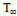. After the time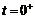, the plate applies a time dependent shear stress f(t) to the fluid along the x-axis. The fluid, together the plate, starts to rotate about z-axis with a constant angular velocity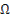and the plate temperature is raised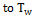. Also, since the plate is infinity in x and y directions all physical quantities depend on z and t only. Therefore, from the continuity equation and condition w (0, t) = 0 it results w=0 everywhere in the fluid. Under the usual Boussinesq’s approximation, the unsteady flow is governed by the following set of partial differential equations [5, 18, 19].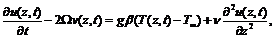(1)(2)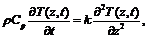(3)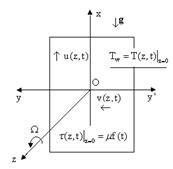Fig. 1. Geometry flow.

where (u(z, t), v(z, t)) are the velocity components along the x-axis and y-axis respectively, g-the gravitational acceleration, β-the coefficient of volume expansion, ν-the kinematic viscosity, ρ-the constant density of the fluid, Cp-the specific heat at constant pressure, k- the coefficient of thermal conductivity and T(z, t) is the temperature of the fluid. Generally, the energy equation contains terms corresponding to viscous dissipation. The fluid is assumed to be purely viscous and the thermal properties are assumed to be constant. For static fluid, dissipation and convective terms become negligible. So, in most situations, we can consider the viscous dissipation term to be zero, with negligible error. Some exceptions may occur when highly viscous fluids are subjected to large velocity gradients (for example, in the polymers processing, the viscous heating is important). The set of equations (1)-(3) is considered together the following initial and boundary conditions: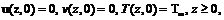(4)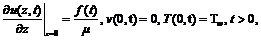(5)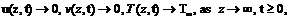(6)

where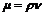is the dynamic viscosity of the fluid and the function f(t) satisfies f(0)=0.

In order to obtain the dimensionless governing equations, we introduce the dimensionless parameters: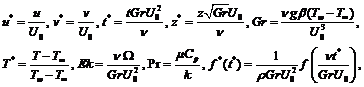where Pr is the Prandtl number, Gr is the Grashof number, Ek is the Ekman number and U0 > 0 is a characteristic velocity. Dropping star notations, we obtain the set of non-dimensional partial differential equations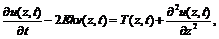(7)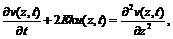(8)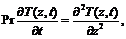(9)

with the initial and boundary conditions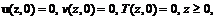(10)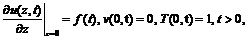(11)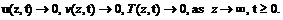(12)

The dimensionless temperature and the surface heat transfer rate respectively are given by , .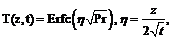(13)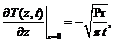(14)

where Erfc(.) is the complementary error function of Gauss. In order to obtain the velocity field, we use the complex velocity field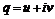and the notation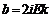q(z,t) is the solution of the problem: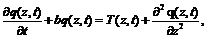(15)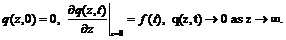(16)

Applying the Laplace transform to Eq. (15) [20-22] and bearing in the mind the corresponding initial conditions for q (z, t), we have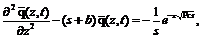(17)

where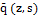is the Laplace transform of the function q (z, t) . The corresponding boundary conditions (16)2,3 become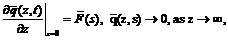(18)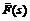being the Laplace transform of the function f(t). The solution of equation (17) subject to the conditions (18) is given by,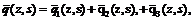(19)

where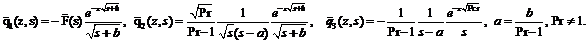(20)

Using the function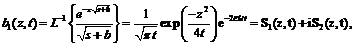(21)

with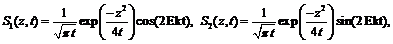(22)

and applying the convolution theorem, we have the inverse Laplace transform for the first term of Eq. (20), namely,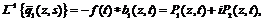(23)

Where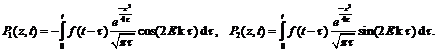(24)

For the second term from Eq. (20), we denote by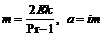, and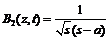.

The inverse Laplace transform of function B2 (z, s) is given by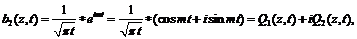(25)

with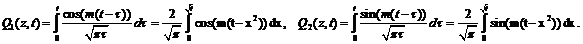(26)

We get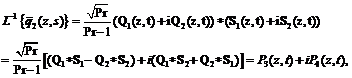(27)

with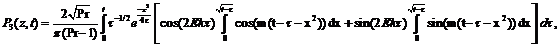(28)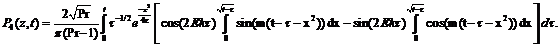(29)

The inverse Laplace of the third term,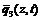is given by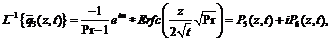(30)

where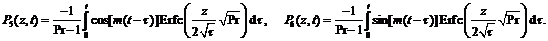(31)

Now, we find the velocity components u(z,t) and v(z,t) as the real and imaginary parts of the complex velocity field, respectively: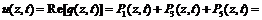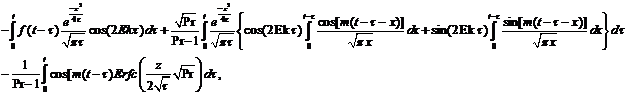(32)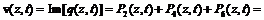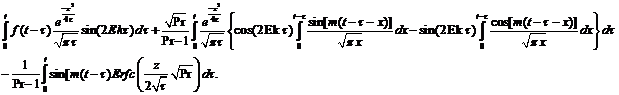(33)

2.1. Particular Cases Ω = 0 (Non-Rotating Frame)

In this case the Ekman number becomes zero, therefore, a = b = m = 0 and

P2=P4=P6=0,                                                                                        (34)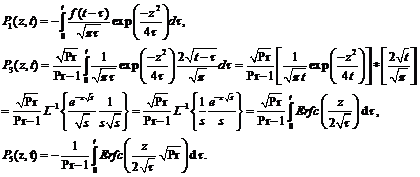(35)

Finally, the velocity components are given by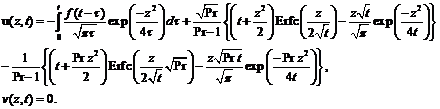(36)

2.2. f(t)=H(t) – the Heaviside Step Unit Function (Constant Shear Stress on the Plate)

Making in Eq. (24) f(t) = 1, the functions P1(z,t) and P2(z,t) become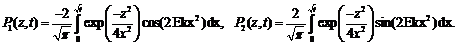(37)

The velocity components u(z,t) and v(z,t) are given by Eqs. (32) and (33) in which the functions P1(z,t) and P2(z,t) are replaced by the expressions given in Eq. (37).

3. Numerical Results and Discussion

In order to obtain some information regarding the fluid behavior, numerical calculations have been made. The graphs corresponding to the velocity components u(z,t) and v(z,t) were plotted in Figures 2 - 4 for f(t) = H(t) – the Heaviside step unit function, meaning for constant shear stress on the plate. To verify the accuracy of the numerical calculations, we used the expressions (32) and (33) of the velocity components and the Stehfest's numerical algorithm for calculating the inverse Laplace transform to retrieve the solution in the time domain . The Stehfest’s algorithm is applied to the function given by Eqs. (19) and (20). We denoted by u(z,t) , v(z,t) the values of the velocity components given by Eqs. (32) and (33), respectively, by u1(z,t), v1(z,t) the values of the velocity components given by the Stehfest’s algorithm, namely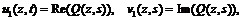(38)

where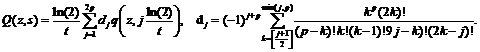(39)

In the above relation, [r] denotes the integer part of the real number r. Table 1 contains the absolute errors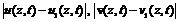for p = 11, t = 2, Ek = 0.85 and Pr = 15. From the Table 1, it results that the values obtained by both formulae are in a good agreement.

To analyze the influence of the Ekman number on the fluid behavior, the Fig 2 was presented. This figure shows graphs of the velocity components u(z,t) and v(z,t), versus the spatial coordinate z, for t = 2, Pr = 15 and for three values of the Ekman number. From these graphs we see that, if the values of Ekman number increase, then the thickness of the velocity boundary layer decreases, therefore, if the angular velocity of the frame increases, the thickness of the velocity boundary layer is lower. The aim of the Fig.3 is to present the influence of the Prandtl number on the fluid velocity. It can see that the influence of the Prandtl number is similarly with the influence of Eckman number, meaning that the increasing of the values of Prandtl number leads to the decreasing of the thickness of the velocity boundary layer.

However, in the studied cases, must to observe that the velocity components tend to zero faster for the variable Ekman number than for the variable Prandtl number. For example, u(z,t) becomes zero for z > 3.2 if Ekman number is variable, while u(z,t) becomes zero if z > 5 for variable Prandtl number.

In Fig. 4 are sketched graphs of the velocity components u(z,t) and v(z,t) for three different values of the time t. It is obvious that the fluid motion is stronger in the direction of the y-axis than in the direction of the x-axis, namely, the component u (z, t) becomes zero if z > 2.5 while the component v (z, t) becomes zero if z > 5.

Table 1. Absolute errors of the velocity components.

z |u(z,t)-u1(z,t)|| v(z,t)-v1(z,t)|

 0 1.63-10-4 1.976-10-4 0.05 1.074-10-4 5.634-10-4 0.1 1.1-10-4 7.673-10-4 0.15 1.205-10-4 5.443-10-4 0.2 1.753-10-4 5.09-10-4 0.25 1.588-10-4 5.001-10-4 0.3 2.031-10-4 5.262-10-4 0.35 1.496-10-4 4.175-10-4 0.4 1.362-10-4 3.455-10-4 0.45 1.598-10-4 3.873-10-4 0.5 1.277-10-4 4.946-10-4 0.55 1.583-10-4 2.412-10-4 0.6 1.415-10-4 3.568-10-4 0.65 1.827-10-4 3.985-10-4 0.7 2.211-10-4 2.992-10-4 0.75 1.748-10-4 2.801-10-4 0.8 2.048-10-4 3.331-10-4 0.85 1.976-10-4 2.763-10-4 0.9 1.798-10-4 3.314-10-4 0.95 1.694-10-4 3.378-10-4 1 1.874-10-4 3.274-10-4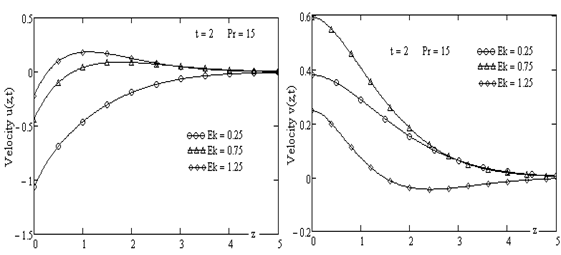Fig. 2. Velocity components u(z,t) and v(z,t) for the variable Ekman number.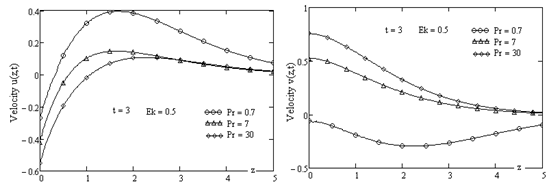Fig. 3. Velocity components u(z,t) and v(z,t) for the variable Prandtl number.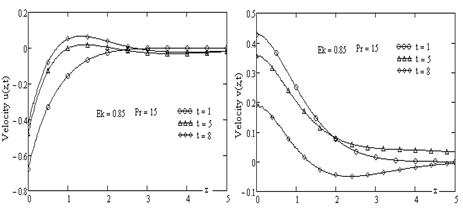Fig. 4. Velocity components u(z,t) and v(z,t) for the variable time t.

4. Conclusions

In this paper, the unsteady flow of an incompressible, homogeneous Newtonian fluid past an isothermal vertical plate in a rotating frame was studied. The governing equations of the flow, together with initial and boundary conditions were written in the non-dimensional form. By means of the Laplace transform method the closed forms of the velocity component in the x- direction u(z,t), respectively in the y-direction v(z,t) were determined. It should be noted that, sometimes, the obtaining of the inverse Laplace transforms by means of the complex analysis techniques can be a difficult process. In such cases the numerical algorithms for the determining of the inverse Laplace transforms are welcome. From this reasons, in this paper we used the Stefhest’s algorithm for the calculating of the inverse Laplace transforms. The analytical solutions and the numerical solution were compared and, the obtained results are in a good agreement. Based on the obtained solutions and using some graphical illustrations generated with the Mathcad software, the influences of Ekman number and Prandtl number on the velocity field were analyzed. It is obtained that, the faster frame rotations reduce the thickness of moving fluid layer. If the values of the Prandtl number increase then, the thickness of the velocity boundary layer decreases. It is important to point out that, in the studied cases, the fluid motion is stronger in the direction of the y-axis than in the direction of the x-axis, namely, the component u (z, t) becomes zero faster than the component v (z, t).

In the most studies of the flows in rotating frame, the boundary conditions refer to the velocity on the boundary or, to the velocity and his derivative in the case of the slip phenomena. In the present paper, is given the shear stress on the boundary. From this reasons, our solutions can be compared with other solutions from the literature by numerical calculations. So, some information about fluid behavior in various boundary conditions can be found. For example, comparing our solutions with solutions given in  with the velocity on the boundary and t > 1, we get that the boundary layer thickness is smaller if the shear stress is given on the boundary.

Acknowledgements

The authors are highly thankful and grateful to the Department of Mathematics, University of Management and Technology, Lahore, Pakistan for supporting and facilitating the Research work.

References

1. A. S. Gupta, I. Pop, V. M. Sounalgekar , Free convection effects on the flow past an accelerated vertical plate in an incompressible dissipative fluid , Rev. Roum. Sci. Techn. Mec. Apl.24, (1979) 561-568.
2. N.G. Kafousias and A .A. Raptis, Mass transfer and free convection effects on the flow past an accelerated vertical infinite plate with variable suction or injection, Rev. Roum. Sci. Techn. Mec. Apl. 26, (1986)11-22.
3. P. Chandran, N. C. Sacheti, Ak. Singh, Natural convection near a vertical plate with ramped wall temperature, Heat Mass Trans.41, (2005) 459- 464.
4. U. S. Rajput, S. Kumar, Rotation and radiation effects on MHD flow past an impulsively started vertical plate with variable temperature, Int. Journal of Math. Analysis, 5(24), (2011) 1155 – 1163.
5. V.M. Soundalgekar, Free convection effects on the Stokes problem for an infinite vertical plate, J. Heat Trans , (Tr. ASME) , 99 , (1977) 499-501.
6. S. Asghar, Masood Khan, A. M. Siddiqui, T. Hayat, Exact solutions for magnetohydrodynamic flow in a rotating, Acta Mech. Sinica 18 (3), (2002), 244-251.
7. S. Abelman, E. Momoniat, T. Hayat, Steady MHD flow of a third fluid in a rotating frame and porous space, Nonlinear Analysis: Real World Appl., 10 (2009), 3322-3328.
8. T. Hayat, Liaqat Ali Khan, R. Ellahi, S. Obaidat, Exact Solutions on MHD Flow Past an Accelerated Porous Plate in a Rotating Frame, Chinese Physics Letters, 28(5), 2011, 054701. doi:10.1088/0256-307X/28/5/054701.
9. T. Hayat, S. Abelman, M. Hamese, Oscilatory Couette flow of rotating Sisko fluid, Appl. Math. Mech.-Engl. Ed. 35 (10) (2014), 1301-1310.
10. M. Kumari, G. Nath, Transient rotating flow over a moving surface with a magnetic field, Int. J. Heat Mass Transfer, 48, (2005) 2878 – 2885.
11. R. Muthucumaraswamy, N. Dhanasekar, G. E. Prasad, Rotation effects on unsteady flow past an accelerated isothermal vertical plate withvariable mass transfer in the presence of chemical reaction of first order, J. Appl. Fluid Mech., 6 (4), (2013) 485 – 490.
12. M.A. Imran, D. Vieru, I. Mirza, The influence of Ekman number on flows over an oscillating isothermal vertical plate in a rotating frame (JAFM in press (2014)).
13. N. D. Waters, M. J. King, Unsteady flow of an elastico-viscous liquid , Rheol. Acta. 9(3), (1970) 345-355.
14. D. Vieru, C. Fetecau, A. Sohail, Flow due to a plate that applies an accelerated shear to a second grade fluid between two parallel walls perpendicular to the plate, Z. Angew. Math. Phys. 62, (2011) 161-172.
15. C. Fetecau, D. Vieru, C. Fetecau, Effect of side walls on the motion of a viscous fluid induced by an infinite plate that applies an oscillating shear stress to the fluid, Cent. Eur. J. Phys. 9, (2011) 816 - 824.
16. C. Fetecau, C. Fetecau, M. Rana, General solutions for the unsteady flow of second-grade fluids over an infinite plate that applies arbitrary shear to the fluid, Z. Naturforsch. 66a, (2011) 753 - 759.
17. N. Ahmed, S. Talukdar, Transient magnetohydrodynamic (MHD) flow of a visco-elastic fluid past an infinite vertical porous plate embedded in a porous medium with Hall current and slip condition in a rotating system, Int. J. of Physical Sciences 7(45), (2012) 5942-5953.
18. A. Q. Mohamed, I. Khan, Z. Ismail, S. Shafie, The unsteady free convection flow of second grade fluid in rotating frame with ramped wall temperature, AIP Proceedings 1605, 398 (2014), doi:10.1063/1.4887622.
19. C. Fetecau, . Vieru, Corina Fetecau, S. Akhtar, General solutions for magnetohydrodynamic natural convection flow with radiative heat transfer and slip condition over a moving plate, Zeitschrift Naturforshung A, 68a, (2013) 659 -667.
20. A. P. Prudnikov, Y. A. Brychkov, I. O. Marichev, Integrals and Series, vol. 4: Direct Laplace Transforms, Gordn and Brech, New York, (1992).
21. A. P. Prudnikov, Y. A. Brychkov, I. O. Marichev, Integrals and Series, vol. 5: Inverse Laplace Transforms, Gordn and Brech, New York, (1992).
22. R. B. Hetnarski, An algorithm for generating some inverse Laplace transform of exponential form, J. Appl. Math. Physics (ZAMP), 26, (1975) 249-253.
23. H. Stehfest, Algorithm 368: Numerical inversion of Laplace transforms, Communication of the ACM 13(1), (1970) 47-49.

 Contents 1. 2. 2.1. 2.2. 3. 4.
Article ToolsAbstractPDF(257K)